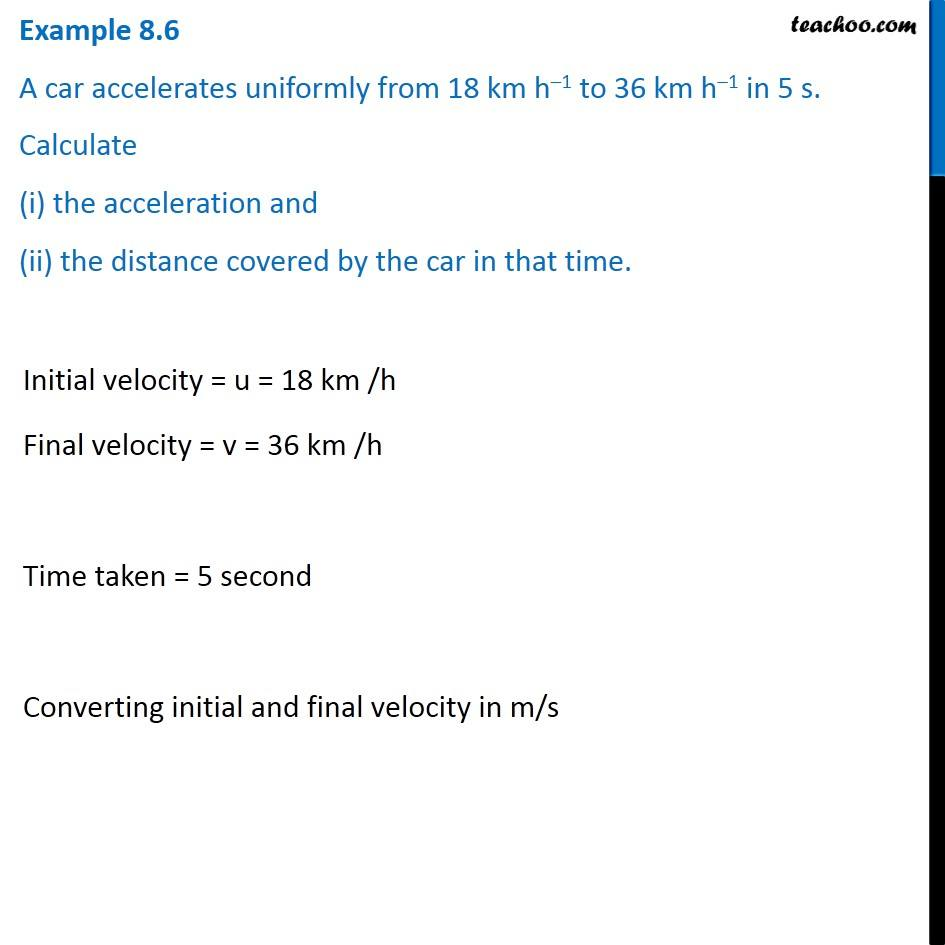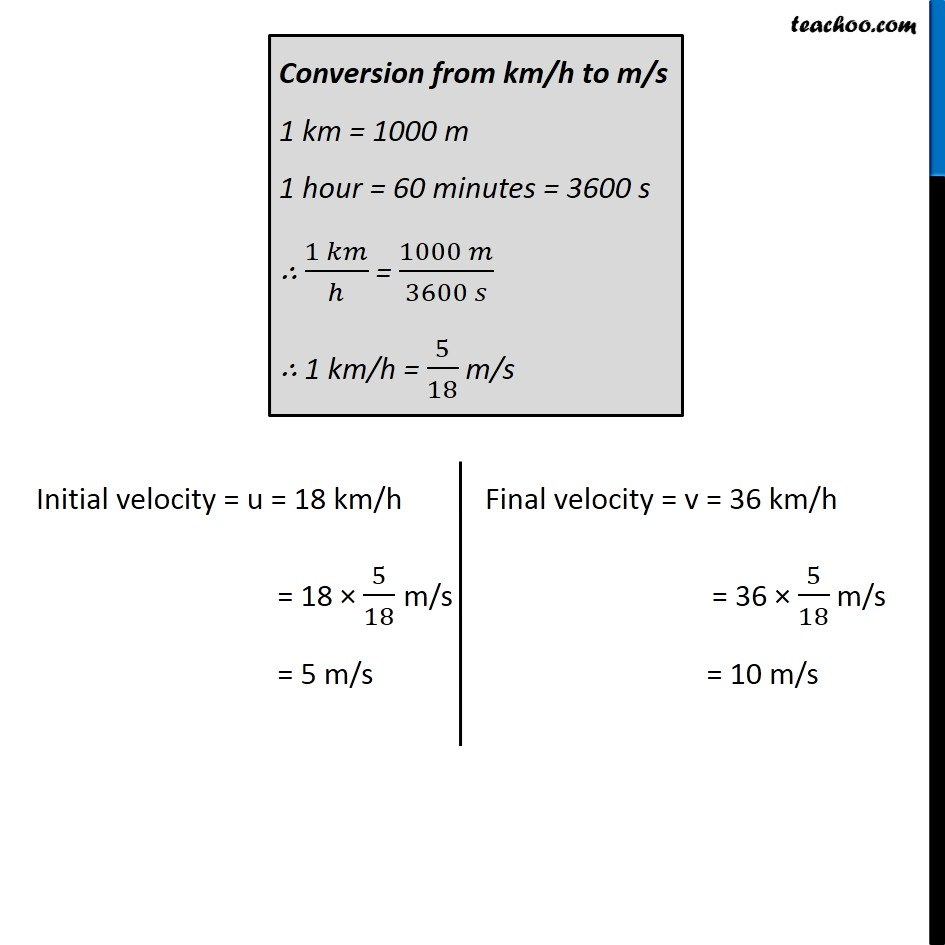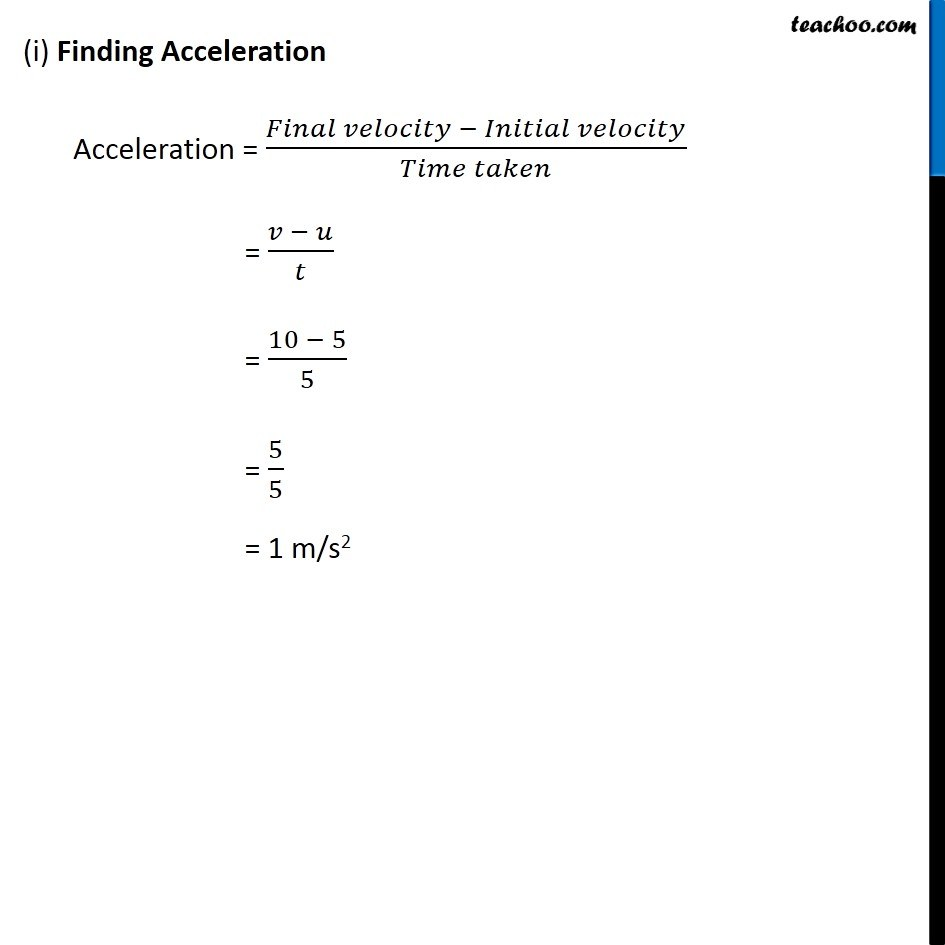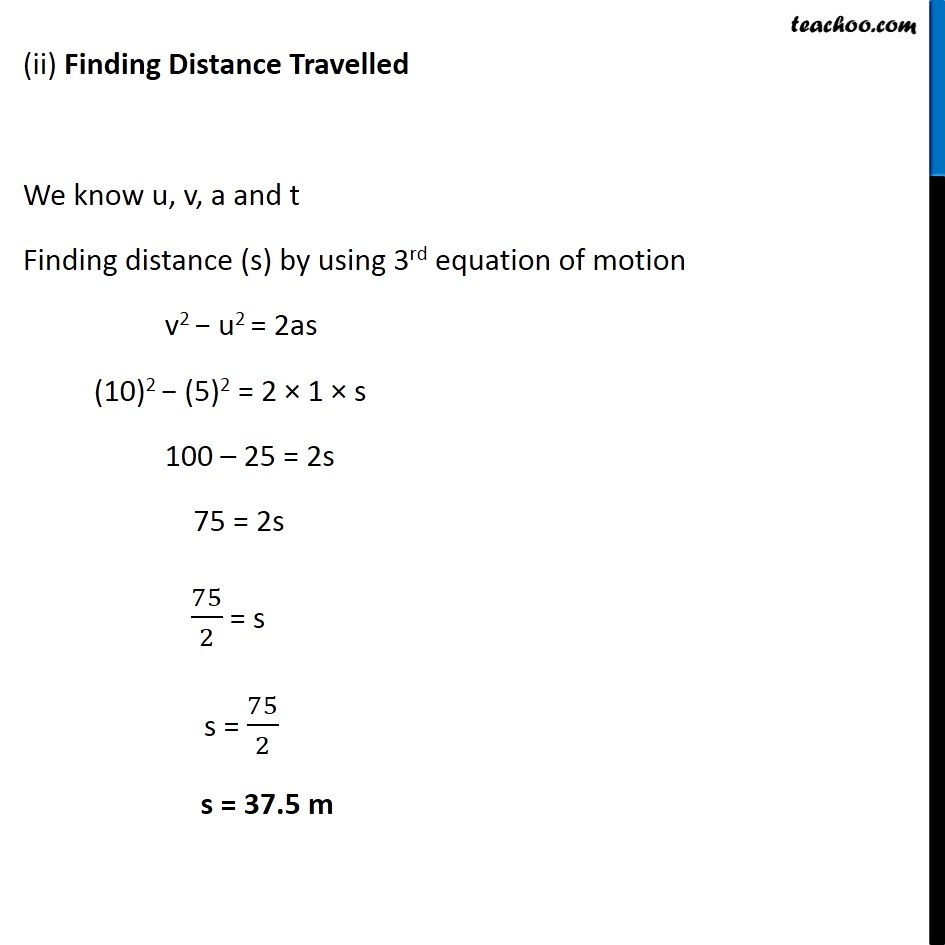Examples from NCERT Book

Class 9
Chapter 8 Class 9 - MotionLearn in your speed, with individual attention - Teachoo Maths 1-on-1 Class

### Transcript

Example 8.6 A car accelerates uniformly from 18 km h–1 to 36 km h–1 in 5 s. Calculate (i) the acceleration and (ii) the distance covered by the car in that time. Initial velocity = u = 18 km /h Final velocity = v = 36 km /h Time taken = 5 second Converting initial and final velocity in m/s Conversion from km/h to m/s 1 km = 1000 m 1 hour = 60 minutes = 3600 s ∴ (1 𝑘𝑚)/ℎ = (1000 𝑚)/(3600 𝑠) ∴ 1 km/h = 5/18 m/s Initial velocity = u = 18 km/h = 18 × 5/18 m/s = 5 m/s Final velocity = v = 36 km/h = 36 × 5/18 m/s = 10 m/s (i) Finding Acceleration Acceleration = (𝐹𝑖𝑛𝑎𝑙 𝑣𝑒𝑙𝑜𝑐𝑖𝑡𝑦 − 𝐼𝑛𝑖𝑡𝑖𝑎𝑙 𝑣𝑒𝑙𝑜𝑐𝑖𝑡𝑦)/(𝑇𝑖𝑚𝑒 𝑡𝑎𝑘𝑒𝑛) = (𝑣 − 𝑢)/𝑡 = (10 − 5)/5 = 5/5 = 1 m/s2 (ii) Finding Distance Travelled We know u, v, a and t Finding distance (s) by using 3rd equation of motion v2 − u2 = 2as (10)2 − (5)2 = 2 × 1 × s 100 – 25 = 2s 75 = 2s 75/2 = s s = 75/2 s = 37.5 m